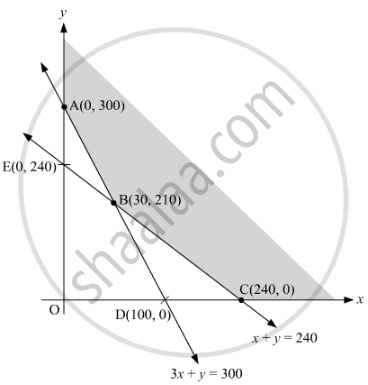# There are two types of fertilisers 'A' and 'B'. 'A' consists of 12% nitrogen and 5% phosphoric acid whereas 'B' consists of 4% nitrogen and 5% phosphoric acid. After testing the soil conditions, farmer finds that he needs at least 12 kg of nitrogen and 12 kg of phosphoric acid for his crops. If 'A' costs Rs 10 per kg and 'B' cost Rs 8 per kg, then graphically determine how much of each type of fertiliser should be used so that nutrient requirements are met at a minimum cost - Mathematics

There are two types of fertilisers 'A' and 'B'. 'A' consists of 12% nitrogen and 5% phosphoric acid whereas 'B' consists of 4% nitrogen and 5% phosphoric acid. After testing the soil conditions, farmer finds that he needs at least 12 kg of nitrogen and 12 kg of phosphoric acid for his crops. If 'A' costs Rs 10 per kg and 'B' cost Rs 8 per kg, then graphically determine how much of each type of fertiliser should be used so that nutrient requirements are met at a minimum cost

#### Solution

 Fertilizer Nitrogen Phosphoric Acid Cost/kg (in Rs) A 12% 5% 10 B 4% 5% 8

Let the requirement of fertilizer A by the farmer be x kg and that of B be y kg.

Given that farmer requires atleast 12 kg of nitrogen and 12 kg of phosphoric acid for his crops.

The inequations thus formed based on the given problem will be as follows:

12/100x + 4/100y ≥ 12

12x + 4y  1200

3x + y  300                  .....(1)

Also,(5x)/100 + (5y)/100≥12

5x + 5y  1200

x + y  240                 .....(2)

x0 and y0          .....(3)

Total cost of the fertilizer Z = 10x + 8y

To solve the LPP graphically, we convert the inequations into equations to obtain the following lines:
3x + y = 300
x + y = 240
x = 0
y = 0

Based on the above equations the graph obtained will be:Corner points Z A (0, 300) 2400 C (240, 0) 2400 B (30, 210) 1980

From the table, it is clear that the minimum value of Z is obtained at C(30, 210). The minimum value of Z is 1980.So, the minimum requirement of fertilizer of type A will be 30 kg and that of type B will be 210 kg.

Concept: Graphical Method of Solving Linear Programming Problems
Is there an error in this question or solution?
2015-2016 (March) All India Set 2 C

Share